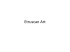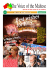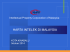# Carmel Vassallo

## Transcription

Carmel Vassallo
```THE
BRIGANTINE T R A D E IN X V I I I
CENTURY MALTA
Carmel Vassallo
A n t h o n y Luttrel] has stated that w e l i v e in " a n a g e w h e n social and e c o n o m i c
rather than p o l i t i c a l , r e l i g i o u s o r c u l t u r a l topics are at the centre o f the h i s t o r i a n ' s
concern"'. B u t as he goes o n to point out, this has not been the case in M a l t a ' s
historiography.
Nearly 3 5 years after Clarke Weber's call f o rresearch into a subject a s
critically i m p o r t a n t for M a l t a astrade w e are not that m u c h nearer to k n o w i n g
the answers t osome o fthe questions h eposed, such a s" . . . h o w important was
M a l t a in regard to trade w i t h the ports o fS o u t h e r n E u r o p e , N o r t h e r n A f r i c a and
the Near East? W h a t were the principal routes of trade used and the chief articles
o f c o m m e r c e e x c h a n g e d ? W h e n d i d t h i s c o m m e c i a l a c t i v i t y r e a c h its p e a k a n d
w h y did itsubsequently decline?":. Clarke Weber believed w e had t o answer
these q u e s t i o n s i f w e are to a r r i v e at a c o m p r e h e n s i v e h i s t o r y o f o u r a r c h i p e l a g o .
T h i s lack o f response to C l a r k e Weber's call i sreflected, for example, i n the
collection o f studies o n early m o d e r n M a l t a published this year entitled
edited by V i c t o r M a l l i a - M i l a n e s ' . In contrast
t o f i v e w h o l e p a g e s o f r e f e r e n c e s u n d e r t h e h e a d i n g A r t i n t h e i n d e x t h e r e is o n l y
o n e single reference to trade. T h i s is. o f course, n o o m i s s i o n o n the editor's p a n
b u t s i m p l y a reflection o f t h e a b s e n c e o f b a s i c research i n t o t h e i s l a n d ' s e c o n o m i c history in the m o d e r n period. Indeed, the solitary efforts b yJ o h n D c b o n o and t o
s o m e extent by Victor M a l l i a - M i l a n e s h i m s e l f o n l y serve to highlight the paucity
o f published studies in this field.
Hospitaller Malta 1530 -1798,
O u r original brief had been the study o f Maltese merchants present in Spain
in the second h a l f o f the X V I I I C e n t u r y , a presence w h i c h b y all accounts w a s
1. A L u l t r e l l " E i g h t e e n t h C e n t u r y M a l t a . P r o s p e r i t y
Malta 1982
and Problems",
i n H y p h e n . V o l . I l l , N° 2 .
p.37
2. B . Clarke Weber
w r i t i n g " , i n Mrlim
"The
Hislory o fMalta. 1500 • 1798
Hinnrira
Vol
3 . V . M n l l i a - M i l a n s l e d ] Hospitaller
Some
opportunities for research
I I . N * 3 . M a l t a 1958 p.1 4 1 .
Malta
1530 • I7VX. M a l t a
1993.
and
108
Cannel Vassal to
inXVIil
Century
Malta
109
inextricably lied t othe export o fcotton y a m t othe Iberian Peninsula. B u t this
traditional picture o fa p r i m a r y product-based export economy has had t o b e
shall limit ourselves to exploring jusi one, although w e believe the most critical,
sector of M a l t a ' s c o m m e r c e in the
Century: the brigantine trade to Spain
and Portugal.
A study o f p r e v i o u s l y unpublished data w o u l d seem t oindicate, amongsL
other things, that the cotton trade was not w h a t ithad been made out to b e and
t h a i M a l t a ' s p r e m i e r e x p o r t for m o s t o f the X V I I I C e n t u r y w a s i n fact capital.
T h e presence o f Maltese merchants i n S p a i n w a s the reason for the cotton exports
a n d not the reverse. T h e s e totally unexpected conclusions led the author deeper
and deeper into uncharted territory o fMaltese c o m m e r c i a l hisiory and pointed
to the urgenl need t o seek concrete answers t o the general queslions posed b y
Clarke Weber.
In the
documentation relating to 1746 there are 842 mentions o f
vessels o fa r o u n d 25 different types a r r i v i n g in M a l t a . T h e largest p r o p o r t i o n o f
these, or 2 0 % , w e r e
and a l t h o u g h s o m e o f these w e r e r e t u r n i n g f r o m
as far a w a y as G e n o a a n d M a r s e i l l e the m a j o r i t y w e r e fairly s m a l l craft w h i c h
did not even warrant a name. T h e y typically had a complement o faround 6 and
were mostly engaged in femying mail, people and very small quantities o f cargo
to a n d f r o m n e a r b y S i c i l y , i n fact the p r o x i m i t y o f the d e s t i n a t i o n m e a n t that t h e
s a m e boat, identifiable b y the n a m e o f its skipper, w o u l d d o a n u m b e r o f trips
d u r i n g the course o f a year. A n e x t r e m e e x a m p l e o f this p h e n o m e n o n w a s the
o r m a i l boat, skippered b y A n g e l o D i a c o n o w h i c h
w o u l d cross over to Sicily once o rtwice a m o n t h . A s a consequence o fthis the
number of
i n a r r i v a l / d e p a r t u r e registers is " i n f l a t e d " .
XVTII
T h e k e y t o an understanding o f M a l t a ' s trade in the
Century must
surely lie in the
( C M ) section o f the National Archives o f
M a l t a ( N A M ) , located a t M d i n a ' s
and other important
d o c u m e n t s t ob e f o u n d a i the N A M ' s principal premises i n Rabat, the S a n t o
Spirito Hospital. Together w i t h documents already k n o w n t ohistorians, in the
Order' o w n archives at the National Library o f M a l l a ( N L M ) , there is e n o u g h
m a t e r i a l t o o b t a i n a n o v e r a l l p i c t u r e o f M a l t e s e t r a d e i n t h e XVm
Century.
Consolato di Mare
Banca Giuratale,
A considerable a m o u n t o f material has already been gathered towards this
e n d a n d a l t h o u g h itis still being processed and studied, it isalready possible t o
p e r c i e v e that a t least s o m e o f the c o n c l u s i o n s w h i c h w i l l e m e r g e w i l l n o t b e a t
all congruent w i t h traditional perceptions o f o u r history i n the m o d e r n period.
H o s p i t a l l e r M a l t a ' s m u c h vaunted e n m i t y to Islam, for example, w a s n o obstacle
to f l o u r i s h i n g c o m m e r c i a l relations w i t h the O t t o m a n E m p i r e 1 . I n this essay w e
4.
B r i a n B l o u e t has already h i n t e d a t h o w "there are. in general, ralher m o r e references
between
M a l t a a n d M o s l e m states than w o u l d b eanticipated c o n s i d e r i n g the c r u s a d i n g
o f t h e O r d e r " ( B . B l o u e t . The Story
of Malta.
of T u n i s in the X V I I and X V I I I Centuries respectively
n e l M a g reb B a r b a r e s c o ( S e c o l i X V I
relations
w i t h the Regency
(S. B o n o " G u c t r a C o i s a r a e C o m m e r e i o
- X I X ) " . i n ElComert;
A fternaliu.
Corsarisme
i
- 3 eTrimestre. 1963
B u t the total o f45 voyages between
60 years, between
Conlraban
Prattiche
speronare
Speronara del Dispaccio.
speronare
T h i s criticism i s probably also applicable t othe next most-frequently
encountered craft in 1746. the tartan. T h e r e were 1 4 0 m e n t i o n s o f these, o r 1 7 %
o f the lotal n u m b e r , e n g a g e d i nferrying huge quantities o f corn, m o s t l y f r o m
nearby S i c i l y . W e are still in the progress o f studying the cargoes but these vessels
carried u p to 1,300
o f g r a i n as w e l l as coal a n d t i m b e r . T h e y h a d c r e w s o f
up to a couple o fdozen men.
salme
T h e third m o s t p r o m i n e n t lype o f ship i n 1746, and the one w eare concerned
w U h t o d a y w a s the b r i g a n t i n e . W e e n c o u n t e r 117 m e n t i o n s o f these, o r 1 4 % o f
the total, i n this year. T h e m a j o r i t y carried a c o m p l e m e n t o fa r o u n d 6 t o 1 2
people and w e r e engaged m a i n l y i nthe carriage o f large quantities o f w i n e a s
w e l l as c h e e s e ,
p o u l t r y , b e a n s , o i l , fish, h o n e y , l i v e s t o c k a n d s n o w . O n l y
o n e brigantine carried grain i n 1746. A si n the case o fthe tartans m e n t i o n e d
a b o v e the trade was m a i n l y carried on w i t h Sicily.
ricotta,
A breed apart were the brigantines w i t h crews o f a couple o fdozen w h i c h
went t oLeghorn, Genoa, Marseille but most o fall, to Spain and Portugal.
1 9 9 0 pp 141 - 1 4 2 a n d L . Valensi
" L e s relations Comitiereiales entre l a R c g c n c c d eT u n i s e l M a l t e au X V I I le Siecle". in
N"43
nature
M a l t a 1992 p.1171. O t h e r authors, such a s B o n o
a n d V a l e n s i . h a v e g i v e n specific details c o n c e r n i n g M a l t a ' s e c o n o m i c
de Tunisia
XVIII
Caliten
passim).
Malta and Tunisia given b yValensi for a period o f nearly
1740 and 1798. averaging
less t h a n o n e per year, i s d w a r f e d b y t h e t o t a l o f
ePassegieri.
a r o u n d 61 for just one year. 1746. w i t h T u n i s i a a n d other O t t o m a n and N o r t h A f r i c a n ports. I n
traffic w a s carried o n via non-Maltese
fact o n l y o n e o fthese 6 1 s h i p s c a m e f r o m T u n i s c o m p a r e d
registered
di Barheria. 5 from Negropnnte,
with 9 from Canea. 8from Tripoli
4 from S m i r n e . and others f r o m a dozen
o d d ports i n the
O t t o m a n E m p i r e ( N A M . Santo Spirito. Unclassified. Libretti delle Prattichede Bastimenti con
Gennaro 1746 - Dicembre
1746). T h e cargoes o F 2 2 o f
Ihese ships w e r e b o u n d for other potts but the majority were apparently sold locally. M o s t o f the
under foreign
flags.
shipping but there were Maltese
Malta's situation m a y have been
p r e s e n c e o fI h e c r u s a d i n g O r d e r o f St .J o h n b u t c e r t a i n e c o n o m i c
re a s s e r t i n g t h e m s e l v e s . A f t e r a l l . b u s i n e s s i s b u s i n e s s .
ships a swell,
heavily conditioned
some
by the
imperatives have a manner o f
C a r m e l Vassal lo
O n l y 1 4brigiintincs, o u t o fa lotal o f 842 entries, returned f r o m Spain i n
1 7 4 6 , but t h o u g h c o n s t i t u t i n g a Liny p r o p o r t i o n o f the total they represented
w h a t w a s probably (he m o s t d y n a m i c sector o f (he M a l t e s e m e r c h a n t m a r i n e for
a considerable part o f the century because i n contrast to
tartans and
other ships o n the short range victualling bade w h i c h w o u l d only b e away f o r
w e e k s at a t i m e , t h e b r i g a n t i n e e x p e d i t i o n s t o S p a i n a n d P o r t u g a l w o u l d t a k e u p
to t w o and even three years.
speronare.
This had important consequences forcrewmembers. shipowners and others
i n v o l v e d i n the trade. A
Citta
e
w h i c h s e e m s to belong to the m i d X V I I I Century, s h o w s 2 6 4
for Cospicua compared to 2 7 4
T h e situation i n Senglea w a s s i m i l a r . I n o t h e r w o r d s less than 2 % o f
the vessels r e t u r n i n g to M a l t a in the m i d d l e o f the X V I I I C e n t u r y accounted for
as m u c h e m p l o y m e n t as the d o z e n s o f s h i p s p l y i n g t h e M a l t a - S i c i l y r o u t e . O w n e r s
o f brigantines fitted for the Iberian Peninsula w o u l d also benefit accordingly
through their ships being chartered for iong periods while financiers, insurers
and others w o u l d belikewise
Ruolo degl' uontini delle
Senglea Cospicua,
Negozianri per
Marinari delle Speronare e Barcareggio
Spagna
perSrcilia'
The Brigantine Trade i nX V I I I
Century
Malta
! I
I
A rare, but rcgretably undated, description o fthe brigantine trade, cited b y
M a l l i a - M i l a n e s , relates h o w " l a diiigenza, e d atlivitil della N a z i o n e fa che n o n
avendo i M a l l e s i d o v e imptegare luiio i l loro denaro, adattino l al o r o industria
alia mercatura e traffico esterno onde conttovamente si f o r m a n o societk d i
mercanli, che constituiscono con le rispettive loro s o m m e , grossi fondi, c h e
affidano a l o r o C o m m i s s i o n a t i . Questi, ollri d e labori, che si f a n n o nell' [sola d i
calzeite, e Berrete di B o o m b a g e (di cui si fa grande raccolta), p o r t a n o pure denaro
effettivo s ucerti Bastimenli detti Bergantini e passando dalla Sicilia, e
costeggtando la s p i a g g i a e l u o g h i d e l l ' Italia, e della F r a n c i a g i u n g o n o a l t e r m i n e
del l o r o d e s l i n o , che vuoleessere la S p a g n a ed i l P o r t o g a l l o , sempre c o m p r a n d o
e rivendendo diversi generi d i Mercanzie: e facendo altretanto nel ritornare a
M a l t a si p o r t a n o accresciuto il fondo, E si fa poi fra i c o m p a r i z o n a r i la d i v i s i o n s
effected.
T h e t e r m brigantine i s nol used here t om e a n a n exact type o f vessel.
N e v e r t h e l e s s , a r e v i e w o f h u n d r e d s o f s h i p s ' i n v e n t o r i e s a n d c o m p a r i s o n of J o s e p h
Muscat's line drawings, reproduced in the appendix, w o u l d seem to point towards
the X V I I I Century Maltese b e r g a n t i n o r
having been some local
adaptation o f w h a t , i n the w i d e r Mediterranean, w e n t under the label xebec,
n a m e l y a t w o o r three m a s t e d boat rigged w i t h lateen sails and equipped w i t h
oars, but it c o u l d also h a v e referred t o o t h e r types o f vessels. I n fact, ships listed
as brigantines o n their departure o rarrival i nM a l t a are referred t ob y other
labels w h e n a r r i v i n g o r d e p a r t i n g f r o m S p a n i s h ports'*. F o r o u r p u r p o s e s t h e r e f o r e
the t e r m brigantine i s above all a generic label used t o describe a c o m p l e x
c o m m e r c i a l operation and not necessarily a specific type o f vessel7.
bergantino
as h a v i n g t w o or three masts, a n d lateen o r a c o m b i n a t i o n o flateen a n d square sails,
depending
on the author.
It m u s t h a v e b e e n t h e s a m e f o r t h e " M a l t e s e " b r i g a n t i n e . W e h a v e e n c o u n t e r e d b r i g a n t i n e s o n
the S p a n i s h trade i n v e n t o r i e d f r o m as little a s 4 0 0 s c u d l f o r a b e r g a n t i n o p i c c o l o I N A M .
( A O ) . V o l . 6 7 Y e a r 1 7 6 6 I n v e n t o r y o f S S C r o c c f i s s o o fA n d r e a Puce) t oa m o r e n o r m a l
of thousand scud]
T h e value o finventories for fully
range f r o m 8 0 0 t o t S O O s c u d l ( N A M , C M , A O Vol
fitted
oul brigantines for Spain i n 1744
2 5Year 1744 passim)
B y the late
a n d early 1 7 6 0 s . a t the height o fthe b r i g a n t i n e Iradc t oS p a i n , the v a l u e o f s h i p and
I7_50s
equipment
w a s n o r m a l l y i nexcess o f2 . 0 0 0 s c u d ) [ N A M . C M . A O Vols. 5 5a n d 5 6f o r 1 7 5 9 a n d
respectively.
C M .
couple
1760
Passim).
T h e lack o f consensus concerning the brigantine is
reflected
i n the m a t t e r o fits a r m a m e n t . E a r l e
believes that the b r i g a n t i n e bad n oc a n n o n a n d w o u l d n o r m a l l y not e v e n have the o n e o r t w o
s w i v e l g u n s f o u n d o n f r i g a t e s I P E a r l e Corsairs
c o n c l u s i o n is based o n a
5. N L M , A r c h i v e s O r d e r o f M a l l a ( A O M )
1067 R u o l o d e g l ' u o m i n i delle Citttt Senglea e Cospicua.
register
of Malta
andBarbary,
L o n d o n 1970 p.54). H i s
o f M a l t e s e s h i p p i n g for the late X V I I C e n t u r y and is c o n f i r m e d
b y o u r analysis o f the 13 brigantines w h i c h iefl M a l t a for S p a i n i n 1699 ( N A M . C M . M u n i f e s t i
B u n d l e I ) T h e s e carried, at m o s t , ac o u p l e o f s w i v e l g u n s or p e r t i e r l . N e v e r t h e l e s s . Ihe s i t u a t i o n
6. L u i s A u d i v e r t . G r a c i o d i C a n d i u a n d Francesco
M a l l i n all let! M a l l a i n 1760 in c o m m a n d o f
b r i g a n t i n e s bur o n a r r i v a l i n S p a i n i h e ships o f t h e first t w o s k i p p e r s w e r e referred t o a s
M a h c s a s " w h i l e the third is
referred
"Fragatas
t oa s " J a b e q u e " ( A r c h i v e G e n e r a l d e S i m a n c a s .
(Spain).
Sectetoria y Superintettdencia d e Hacienda Leg. 1131),
requires
further research. Despite the evident similarity between the xebec and Ihe brigantine in Ins line
drawings. Joseph Muscat has insisted, i nprivate conversations and public discussion, o n the
uniqueness o fthe Maltese brigantines. Nevertheless, the question o fidentifying and
recognize.
comparing
T h e xebec is described
b ythe m i d X V I I I C e n t u r y and despite
Muscat's c l a i m that the X V I I I
brigantine "carried no g u n s " (J. Muscat's Appendix o fLine D r a w i n g s i n A . H . J
of the Sea, M a l l a 1 9 8 9 p p . 1 8 8 - 1 8 9
reviewed
7. W h a t t h e M a l t e s e b r i g a n t i n e c o n s i s t e d o f i n s t r i c t n a u t i c a l t e r m s i s a m a t t e r w h i c h
different types o fvessels is a c o m p l e x issue as m a n y a u t h o r s
refer
Century
P r i n s ' s In
Peril
appendix) most o fthe numerous inventories w e have
f o r the 1740s, 1 7 5 0 s a n d 1760s m a k e m e n t i o n o f at least t w o a n d o c c a s i o n a l l y
even
" Q u a t t r o C u n o n e con leloro Cascte e suoi f o m i m e n t i e con dodici Balle di f e r r o " ( N A M .
C M .
A O , Vol. 51 Year 1756 Inventory o f PadronTomasoAgius's
brigantine) The "Maltese" briganline
a n d its e q u i p m e n t w a s c l e a r l y i n a state o fc o n t i n u a l e v o l u t i o n .
8 , C i t e d i n M a l l i a - M i l a n e s Descrizione
p.55
di Malla
Anno
1716 - A Venetian
Account,
Malta
1988
Carmel Vassal I o
112
It is c l e a r that w e are c o n f r o n t e d w i t h w h a t B r a u d e l has c a l l e d " t r a m p i n g " ,
n a m e l y the l o n g succession o f b u y i n g , s e l l i n g a n d e x c h a n g i n g at ports all a l o n g
t h e c o a s t s o t y p i c a l o f M e d i t e r r a n e a n w a t e r s as far b a c k as c a n b e r e m e m b e r e d " .
B u t the Maltese brigantine had a special characteristic w h i c h .distinguished it
f r o m mere t r a m p i n g and this was the beaching o fthe vessel on arrival in Spain
or Portugal and the transformation o f the sailors into land-based retailers and
pedlars. I n 1745 - 1746, for e x a m p l e , brigantine expeditions t oV a l e n c i a lasted
an average o f 16 m o n t h s ' " . O f this t i m e , a r o u n d 9 m o n t h s w e r e spent in S p a i n
itself compared t o7 m o n t h s gelling t oand f r o m S p a i n " .
T h i s m o s t l y itinerant f o r m o f d o i n g business isw h a t has prevented Maltese
brigantine expeditions f r o m attracting the attention o f Spanish historians. It was,
in fact, o n l y after the a b a n d o n i n g o fthe brigantine i n the second h a l f o f the
c e n t u r y , as a c o n s e q u e n c e o f i n c r e a s i n g o p p o s i t i o n , that M a l t e s e m e r c h a n t s started
to appear in notarial d o c u m e n t s , tax rolls, parish records and o t h e r d o c u m e n t a t i o n
traditionally used b y historians to study sedentary populations. B u t despite this
l o w profile Maltese hrigantine-borne merchants were very important indeed.
I n t h e e a r l y 1 7 6 0 s t h e y c o n s t i t u t e d I i n 10 o f a l l f o r e i g n m e r c h a n t s i n S p a i n
at a t i m e w h e n the latter w a s the g a t e w a y t o the riches o f A m e r i c a a n d w h e n
business was o v e r w h e l m i n g l y in the hands o f foreigners'2.
A n a n o n y m o u s G e r m a n gentleman w h o travelled through Spain in 1764 1 7 6 5 w r o t e t h a t " t h e M a l t e s e d o a l o t o f t r a d e i n C a d i z a n d y o u w o n ' t find a n y
important city all over Spain where y o u cannot find them. T h e y have more
privileges and rights than Spanish shopkeepers w h o o n l y sell s m a l l a m o u n t s . . .
T h e Briganrine Trade i nX V I I I Century
Malta
T h e y take their merchandise f r o m Genoa. Marseille and other ports o f the
Mediterranean. T h e y take everything and arrive w i t h entire loads o f all kinds o f
g o o d s o f w h i c h o n l y a few are f r o m M a l t a . . . a n d take large s u m s o f cash h o m e
from Spain"13.
pesos
T h e l a t t e r p o i n t i s c o n f i r m e d b y o f f i c i a l figures f o r t h e e x p o r t o f s i l v e r
from Spain. I n 1761 and 1762, for example, the Maltese were responsible for
1 4 % and 1 7 % respectively o f official exports o f silver f r o m S p a i n ' J . It w a s a big
T h e o r i g i n o f the trade probably l a yi n the increasing difficulties being
experienced b y the corsair sector in M a l t a w h i c h caused capital and labour t o
seek n e w o u t l e t s . T h i s is reflected, for e x a m p l e , i n t h e a l l - i m p o r t a n t m e c h a n i s m
for the financing o f the expeditions, the type o f ship used and in the geographical
o r i g i n o f t h e c a p t a i n s a n d c r e w s . O t h e r m i n o r details, s u c h as t h e p r e f e r e n c e f o r
n a m i n g the vessel
o r variations thereof, also provide
useful pointers.
Santissimo Crocefisso,
T h e e a r l i e s t figures w e h a v e , f o r t h e l a t e X V I I C e n t u r y , s h o w t h a t t h e
brigantine trade was already w e l l established w h e n the
di
was
c r e a t e d i n 1 6 9 7 . I n 1 6 9 9 , 13 s h i p s , o u t o f a t o t a l o f 3 6 8 d e p a r t u r e s , left f o r t h e
I b e r i a n P e n i n s u l a 1 3 . T h i s o n l y r e p r e s e n t e d 3 . 3 % o f t h e t o t a l h u t , as w e s a w a b o v e ,
this d o e s n o t t a k e i n t o a c c o u n t t h e fact thai m a n y o f the d e p a r t u r e s w o u l d h a v e
consisted o f smaller
shuttling to and f r o m nearby Sicily. In 1711 the
n u m b e r h a d g o n e u p t o 18, r e p r e s e n t i n g 1 4 . 2 % o f the departures for that year1'.
I n 1 7 3 0 there were a tleast 21 expeditions t othe Iberian P e n i n s u l a w h i l e 1 4
Consolato
and the Mediterranean
World
in ihe age of Philip
II.
London
1764 und 1765, beschricben
H a r m i nSpanien in den Jahren
von einem seiner Gesellschuft in: Magazin filrdie neure Historic
und Geographic, angclegt v o n D . A n i o n Friedrich BUsching. Z w e y t c r T h e i l . 2. Autlage.
l972Vol.lpp.l06-IO7.
Hamburg
1769" pp.134 - 135, cited i n W, Von D e n Driesch, D i e auslandischen K a u f l e u t e w a h r e n d d e s
10. N A M . S a n i o S p i r i t o . U n c l a s s i f i e d . L i b r e l l o delle P r n u i c h e . . . O c t o b e r 1 7 4 5 - A p r i l 1 7 4 6 a n d
J u n e t oOct.
Mare
speronaras
13. T r a n s l a t e d f r o m I h e a n o n y m o u s " R e i s e n eines v o m n c h e m e n
9 . F . B r a u d e l The Mediterranean
113
18 J a h r h u n d e r t s i n S p a n i e n u n d ihre Bctciltgung a m Kolontalhundcl, Kiiln/Wien. 1972
p-241.
1746
11. A v e r a g e slay i n S p a i n based o n a s a m p l e consisting o f Ihe f o l l o w i n g expeditions t o Valencia:
Juan A m a u d (1760); Claudio Bus 11763); Balthasar Caruana (1763); Clemente G r i m a
14. B a s e d o nA r c h i v o G e n e r a l d eS i m a n c a s ( S p a i n ) , Secreiaria y S u p e r i n t e n d e n c i a d e H a c i e n d a
(1766);
Legajos 1 1 3 1 , 1 1 3 2 . 1 1 3 3 . 1 2 3 3 . 1 2 9 8 . 1 3 4 3 and 1344. Total exports available in A r c h i v o General
Phelipe M a g r o (1762); Salvador R o m a n o (1762) and A n i o n i o Seichel 11763). Details in Areiii vo
de S i m a n c a s (Spain). Hacienda. D i r e c t i o n G e n e r a l d e Rentas 2 'Retnesa Leg. 491)8 '"Razdn d e
General d e Simancas (Spain). Secretaria y Superintendicia d e Hacienda.
l o que h a i m p o r t a d o e l Y n d u l i o del 3 y 4 por 1 0 0 d e l a e x t r a c c i o n d e plaia desde e la n o
Legajos 1343, 1344 and
Rentas
Generales.
1345.
12. F o r t h e n u m b e r o f M a l l c s e i n S p a i n i n 1 7 6 4 - 1 7 6 6 A r c h i v o H i s t d r i c o N a t i o n a l ( M a d r i d ) .
1 5 . N A M . C M . M a n i f e s t ] , B u n d l e N ° 2 f o r 1 6 9 8 - 1 7 0 1 . T o i a l s f r o m A . M a l l i a Zondadori
Legajos 6 2 9 (I), 629 (2) and 6 2 9 (3) and the A r c h i v o Municipal d eM u r c i a (Spain) Legajo
1516
times (1720-
N * 5 a n d L e g a j o 1534. F o r the total n u m b e r o fforeign m e r c h a n l s i n S p a i n . J. Salas A u s e n s
"Les
p.88
F r a n c a i s e n E s p a g n e d a n s l a S e c o n d a M o i t i e d u X V I l l e S i e c l e " . i n let
F Epnque
modenie
(XVIe
• XVIIe
1750
hasla el d e 1783 inclusive, por quiquenios". Undated and unsigned,
siecles).
Franqais
en
( O u v r a g e C o l l e c t i n . Paris 1990. p. 160.
1722).
and his
B.A. (Hons) History unpublished dissertation. University o fMalta
F.spagneii
1 6 . N A M . C M . M a n i f e s t ! . B u n d l e N° I f o r 1 7 0 8 - 1 7 1 1 . T o t a l s f r o m A . M a l l i a . o p . e l l .
1976
114
Carmel
Vassallo
brigantines c a m e back f r o m there17. T h e all t i m e absolute h i g h seems to have
been 1 7 5 9 w i t h at least 2 4 departures18.
T h e average expedition consisted o f around 8 "companies" totalling
s o m e w h a t over 2 0 m e n . T h e cargo consisted m o s t l y o f cash but also included
miscellaneous i t e m s a n d s o m e cotton. I n 1730 the latter represented, o n average,
under 1 0 % o f the cargo in value. A v e r a g e capital per expedition in 1730 was
around 25,000 scudi".
M o s t o f the purchasing was done i n Genoa and Marseille, where additional
l i n e s o f c r e d i t w e r e secured, b u t o t h e r p o r t s s u c h as M e s s i n a , N a p l e s a n d L e g h o r n
were also used. M o s t o f the selling o n the other hand was done in Spain and
Portugal.
O n l y o n e S c r i v a n o ' s o r p u r s e r ' s b o o k has b e e n e n c o u n t e r e d h u t it e n a b l e s
us to f o l l o w the ship's progress up the Italian Peninsula, along the F r e n c h coast
and d o w n the Spanish littoral all the w a y to Lisbon. Captain Benedetto deCandia's
b r i g a n t i n e e x p e d i t i o n to L i s b o n i n 1 7 4 1 - 1 7 4 4 c a n be seen in the a c c o m p a n y i n g
map. It look an abnormally long three years but another partial record o f a later
e x p e d i t i o n reflects m o r e o r less the s a m e pattern w i t h shorter h o p s o n the o u t w a r d
b o u n d j o u r n e y than o n the return leg2".
T h e cargo o f brigantines returning f r o m Spain seems to have consisted m o s t l y
o f t h e s a m e m e r c h a n d i s e a s h a d b e e n t a k e n o u t i n t h e first p l a c e , n a m e l y c o i n .
T e n o f t h e e l e v e n s h i p s r e t u r n i n g d u r i n g t h e c o u r s e o f 12 m o n t h s i n 1 7 4 5 - 1 7 4 6
brought back an average o f around 33,000 scudi in foreign currency21. T h e
M a l t e s e w e r e capitalist merchants, w h o started w i t h m o n e y acquired goods and
regularly returned with money.
T h e i m p o r t a n c e oF the b r i g a n t i n e trade to t h e I b e r i a n P e n i n s u l a is, i n fact,
b e s t r e f l e c t e d i n t h e figures f o r t h e m o v e m e n t o f c a p i t a l .
17. F o r 1730 N A M . C M . A O
18.
V o l . 2 0 a n d for 1746 Prattiche... cited
above.
N A M . C M . A O V o l . 5 5
19. E s t i m a t e s b a s e d o n 2 1 b r i g a n t i n e s l e a v i n g f o r S p a i n a n d P o r t u g a l i n 1 7 3 0 ( N A M . C M . A O . V o l .
20).
20. F o r the S c r i v a n o ' s book for Decandia's
1741 - 1744 expedition refer N A M . C M , A O V o l . 2 6
Y e a r 1 7 4 6 . D e C a n d i a / F a r r u g i a et S o c . F o r d e t a i l s o f F r a n c e s c o C a s s a r ' s b r i g a m i n e
in 1757 - l 7 5 9 . r e f e r N A M . C M , A O V o l . 5 7
21.
Refernote9
and 66
expedition
C a r m e l V a s s a l 1o
116
D e t a i l e d a n a l y s i s o f b o t t o m r y b o n d , o r c a m b i o m a r i t t i m o . lisls, as w e l l a s
foreign currency exports noted d o w n o nbills o f lading for the period 1757 t o
1765, reproduced in appendix I , s h o w s that M a l l a was investing a n average o f
5 5 0 , 0 0 0 s c u d i p.a. i n t h e I b e r i a n trade. T h i s m u s t b e c o n s i d e r e d a m i n i m u m i n
v i e w o f the loose leaf nature o f the d o c u m e n t a t i o n . T h i s a m o u n t i s nevertheless
equivalent to three limes the value o f corsair prizes in the hectic period o f 1660
- 1662".
I n r e t u r n M a l t a g o t b a c k a n a v e r a g e o f a r o u n d 5 5 5 , 0 0 0 p e s o s p.a. f r o m
Spain alone i n 1761 and 17621'. These figures exclude any s u m s repatriated
f r o m C a t a l o n i a for cotton and are r o u g h l y equivalent to 1,222,000 Maltese scudi,
o r seven t i m e s the value o fcorsiar prizes mentioned above.
Maltese brigantines merchants' exports o f silver f r o m Spain i n 1762,
reproduced i nappendix I I , e v e n exceed the i n c o m e o f the Order's Treasury,
w h i c h stood at 1,073.920 s c u d i in 1767 a n d 8 3 2 , 0 4 9 s c u d i in 1 7 7 1 ,o r the c u s t o m s
v a l u e o f c o t t o n e x p o r t e d o r r c - e x p o t l e d t o S p a i n 16 y e a r s later, i n 1 7 7 8 - 1 7 7 9 ,
w h i c h stood at 1,263,240 s c u d i " .
The Brigantine Trade i nXV1I1 Century
In the l i m i t e d space available I have sought to give a very brief o v e r v i e w o f
just one o f the m a n y areas o f vital importance i n o u r social and e c o n o m i c history
w h i c h have received little or n o attention. I trust that it w i l l serve to h i g h l i g h t the
u r g e n t need for m o r e research based o n p r i m a r y d a t a a n d less d e p e n d e n c e o n
w o m out f o r m u l a s and texts.
I f w e are t ohave w h a t Luttrell has called " acorrect consciousness" o f o u r
past a s a p o i n t o f reference f o r o u r m o d e r n p r o b l e m s w e clearly need m o r e
h i s t o r y a n d less m y t h 2 1 . M o r e a b o u t t h e h i s t o r y o f g r o u p s a n d p e o p l e ' s r e l a t i o n s h i p
w i t h t h e i r e n v i r o n m e n t a n d less a b o u t e v e n t s .
T h e r e can b e n odoubt that trade w a s the island's e c o n o m y response t o the
decline o fIhe corsair sector and the stagnation i n the Order's i n c o m e d u r i n g the
M . Fotitenay " L o s F'entimenos Corsarios en la Periferazidn del Mediierrtmeo en E l Siglo X V U " .
(Areas). M u r c i a 1986 p.118
23. 502,810 a n d 6 0 8 . 4 8 0 pesos ruertes in 1761 and 1762 respectively.
R e f e r f o o t n o t e 13 a b o v e .
24. F i g u r e s f o r t h e i n c o m e o f the O r d e r ' s T r e a s u r y f r o m A . L u t t r e l l op. cit. p.43. C u s t o m s v a l u e o f
conon exported or re-exported i n 1778 - 1779 based o n N A M , Santo Spirito. Unclassified " G r a n
L i b r a d i D o g a n a A n n o T e r z o C C del P r i m o M a g g i o 1778 a T u t t Aprile 1779". A detailed analysis
o f this d o c u m e n t w o u l d seem t o indicate that Luitrell's projections o f earnings f r o m cotton
exports, based o n the figures provided b y J o h n Dcbono. m a y constitute a gross
overestimate
(Luttrell op. cit. p. 46). T h e rationale behind D c b o n o ' s adoption o fthe D o g a n a figures, in the
Ricctta Magislrale
records
himself recognizes these
Aspects
of the cotton
a s a p r o x y for c o t t o n e x p o r t s i sn o t t o t a l l y clear b e c a u s e as
figures
i n c l u d e d r e v e n u e o n b o t h exports a n d i m p o r t s . (J.
1750-1S00
Debono
Debono
Unpublished B. A. (Hons) dissertation. University
o f M a l t a 1976 p.52).
2 5 . F . B r a u d e l Cmliwtion
London 1982
p.406
and Capitalism
15th • 18th Century.
Vol II. The Wheels
of
117
course o f the X V I I I Century. T h e principal branch o f this trade consisted o f
brigantine expeditions t o the Iberian P e n i n s u l a w h i c h brought i n the foreign
e x c h a n g e earnings to offset the c h r o n i c trade deficit arising o u t o fthe 1,000,000
p l u s s c u d i f o o d b i l l a n d set t h e s c e n e f o r t h e later d o m i n a t i o n b y t h e M a l t e s e o f
Spain's cotton markeL
T h i s i s clear v i n d i c a t i o n o f Braudcl's v i e w that l o n g distance trade is the
r e a l l y b i g b u s i n e s s . A s h e p u t s i t ".. . d i s t a n c e a l o n e , i n a n a g e o f d i f f i c u l t a n d
irregular c o m m u n i c a t i o n , created ordinary every d a y conditions f o r
profiteering..."15.
22.
Malta
Commerce.
26.
A . Luttrell op. cit. p.37
IIS
I
.Hill.
I
i l l . .
The
Century
Malta
119
APPENDIX I
APPENDIX I I
Amounts invested in the Iberian TVade 1757 - 1765
MALTESE MERCHANTS EXPORTING
PESOS FUERTES FROM SPAIN IN 1762
( L o a n s r a i s e d o r c a s h o n b i l l s o f l a d i n g , w h i c h e v e r is h i g h e r , i n S c u d i ) .
Year
i nXVIII
TVitnl Strain
1
1 1
'.III
CJ \ L 1 1 1 1
V/ilf^ni'tn/
s
• 1 I -. 1 • •. I t l r
Oilier
Alicame
and Portugal
Alicante
Pesos Fuertes
Caruana,
4,200
Magro,
2,080
11S1
S S U
J J O , l
1758
456,050
197,535
117,295
141.220
1759
785,678
408,105
204,935
172,638
A1m e n a
103,510
Domicio, Francisco and
11A
7 7 6 105
167 687
180 037
1760
493,055
307,295
82,250
1761
665.025
414,565
189,740
60,720
1762
654,620
380,683
151.360
122,577
1763
401,025
204,775
149.775
52,075
1764
377,634
208,812
83.287
85,535
1765
585.877
324.265
12,775
235,237
( S o u r c e : O w n c a l c u l a t i o n s b a s e d o n i h e f o l l o w i n g files: N A M , C o n s o l a t o d i
Mare, A l i i Originali. Vols. 53 and 54; 54; 55: 56 and 57; 58 and 59; 54. 60 and
6 1 ; 6 4 ; 65 and 66; 67 and 68 for 1757, 1758. 1759. 1760. 1761. 1762. 1763.
1764 and 1765 respectively).
1,300
Fiteni, Gregorio
Felix
companions
25.000
Abela, T h o m a s and Joseph
7,000
Bergansonc. Pedro
6,000
Bonec, Cayclano
8,000
Bonichi,
Francisco
34,000
Boselil. Benilo
3,500
Bozeiin.
6,500
Santos
di Candia,
Gracio
Caravana,
Joseph
Cast,itia, Juan
38.000
10.000
40,000
Farrulla, Lorenzo
9,000
Galia, Pablo
5.500
Lia, Joseph
5,000
Lia, Phelix
5,500
Malta,
Francisco
40,000
Mauricio, Maximiliano
6,500
Mifsud, Agustin
4,000
Mifsud. Lorenzo
30.000
120
Carmel
Vassallo
Psinga, Joseph
45,000
Ros, Joachin
35,000
Sopard,
The Brigantine Trade in X V I I I
Century Malta
121
APPENDIX III
8.000
Lorenzo
28,000
Spiteri, Joseph
5,000
Tamboni, Pedro
Zaquia, Miguel
5,000
Angel
Cartagena
4.(10(1
de Borga, Joseph
Malaga
3.500
Mamo.
Francisco
Motril
2,300
Azupart. Miguel
2,900
Grech, Miguel
3.800
Angel
2,900
Schembri, Antonio
Ventura, Joseph
Salobrena
27,000
Arnau, Juan and companions
25,000
Becina,
30,000
Romano,
Valencia
Mateo
40,000
Buxeya, Benito
Zahra, Felix and
50,000
companions
T O T A L
(Sources: A r c h i v o General D e Simancas, Secretaria Y
Hacienda Legajos 1132, 1133, 1233, 1298.1344).
608,480 p e s o s
fuertes
Soperintendencia
De
C H E B E C . T h i s vessel was introduced in ihe M e d i t e r r a n e a n by the B a r b a r y
regencies i n the course o f the 17th century and reached its full efficiency u n d e r
the A l g e r i a n corsairs. It w a s also adopted by the Christian powers, the sail
a r r a n g e m e n t , i t s f e w o a r s b u t a b o v e a l l i t s fine u n d e r w a t e r b o d y g a v e i t g r e a t
s p e e d as w e l l as t h e a b i l i t y t o w i t h s t a n d t h e r o u g h w i n t e r w e a t h e r . M a l t e s e o w n e r s
e m p l o y e d t h e c h e b e c f o r t h e g r a i n c a r r y i n g trade as t h e y c o u l d c a r r y as m u c h as
3 0 0 t o n s c a r g o . T h e O r d e r u s e d i t a s a fighting u n i t n o t o n l y t o g u a r d t h e e n t r a n c e
o f the G r a n d H a r b o u r b u t also f o r the corso. A chebec carried up to 14 s m a l l
guns and m a n y swivel guns. T h e r e were different sizes ofchebccs; the one s h o w n
represents the biggest o f its k i n d . T h e O r d e r introduced these ehebecs a r o u n d
the 1730. T h e i r s h a l l o w draught m a d e it possible to approach shores and attack
helpless merchant ships.
( S o u r c e : L i n e D r a w i n g b y J o s e p h M u s c a t i n A . H . J . P r i n s , In
Malta 1989 p.195)
P e r i l o f the
Sea
122
Carmel
Vassallo
B R I G A N T I N E , 18th century. T h i s type o f vessel was seaworthy and cheap to
build. I t was popular amongst Maltese padroni for three centuries. S u c h a frail
l o o k i n g o p e n boat c o u l d take o n up to 4 0 ton burthen. It carried n o g u n s but its
best w e a p o n w a s its s w i f t n e s s . It w i l l be noticed that the sperone at the b o w s is
r e t a i n e d a l t h o u g h its i m p o r t a n c e d i m i n i s h e d i n the 1 8 t h c e n t u r y . T h e s p e r o n e
w a s a distinguished m a r k o n all lateen rigs.
( S o u r c e : L i n e D r a w i n g by Joseph M u s c a t i n A . H . J . Prins, I n
1989p,188).
Sea.Malta
Peril of the
```

### 76- JDEvans, refcring to cult figures and ornamental objects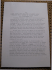### Trade Plumbing memperoleh 1 Medali Perunggu### ultimo MIE:MIE.qxd - Facoltà di Economia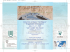### informazioni utili per studenti in visita a malta e gozo per imparare l### Projemato / Metalvolt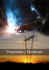### A nove anni da quella data, ricordarli è un dovere### Working Papw No. 247 Isaac - Centre For Development Studies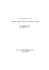### Yth. 1. Para Kepala Kantor Wilayah Direktorat Jenderal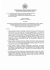### Conservation of Cultural Heritage### catalogo mostra - Provincia di Torino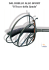### Private equity investments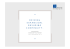### Presentation of AGENCY SIGMA_June_2012 [tryb zgodności]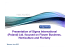### Il supporto finanziario all`interscambio e agli investimenti italiani all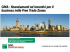### Descargue la lista completa de referencias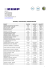### Gate – Mdina - Superintendence of Cultural Heritage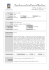### Fare del comparto digitale un elemento chiave del progetto di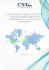### The City of Valletta in an Eighteenth century Map by Sebastiano Ittar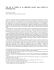### Addendum to Alba Meeting in pdf format### a full CV here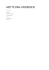### Etruscan Art PDF